## Supreme Theory of Everything: Superconductivity and Non-Ohmic Resistivity in a Negative Singularity of the Open Hysteresis.

Ulaanbaatar Tardad1*, Sangaa Deleg2, Aldarmaa Chuluunbaatar1

1 Department of Physics, School of Applied Sciences. Mongolian University of Science and Technology.

2 Insitute of Physics and Technology, Mongolian Academy of Sciences.

*Corresponding Author:Ulaanbaatar Tardad, Department of Physics, School of Applied Sciences. Mongolian University of Science and Technology.Tel: 976 11 323579; Fax: 976 11 323579; E-mail: tarzad@must.edu.mn

Citation: Ulaanbaatar Tardad, Sangaa Deleg, Aldarmaa Chuluunbaatar (2023) Supreme Theory of Everything: Superconductivity and Non-Ohmic Resistivity in a Negative Singularity of the Open Hysteresis. Nano Technol & Nano Sci J 5: 149.

Received: April 14, 2023; Accepted: April 25, 2023; Published: May 6, 2023.

## Abstract

This article aims to clarify the theoretical formulation of the non-ohmic resistors and superconductors based on the Supreme Theory of Everything (STE). The ultimate goal of the paper focuses on the connections with the next two items. The first item. Physicists have struggled with superconductivity to understand in detail since it was first observed by Heike Kamerlingh Onnes in 1911. Today experimentally confirmed and theoretically predicted that more than 40% of all the chemical elements in the periodic table can be candidates for superconductors. What it means is that not only all the chemical elements and the alloys, but every substance has possible to become superconductors when subjected to extraordinary circumstances (singularity, 0 Kelvin temperature, and diamagnetism). In other words, when the physical conditions are met, the negative singularity of the open hysteresis of the magnetism becomes a black hole and breaks down the atom, producing free electrons, and activating the Cooper pairs and superconductors. Such conditions are already abundant everywhere in the Universe. Cosmological observations indicate that the dominating average temperature is only -270.4°C (2.75K) in all directions in the Universe. The second item. Today, the scientific world goes differentiated into a multitude of low-effective branches. Perhaps, it is time for all scientific, especially physical laws need unite to into one law. This law should not go deep into the detailed fields of science and needs to be based on geometrical methods. In this paper, an alternative theory of superconductivity and nonohmic resistivity is described newly by the trigonometric open hysteresis.

## Keywords

Keywords:Open Hysteresis of Magnetism, Superconductivity, Non-Ohmic Resistors and Critical Temperature.

## Introduction

Ohm’s law is the progenitor of superconductivity. If R equals zero Ohm’s formula (I=U/R) is undefined mathematically. Nonetheless, it is true superconductivity. So, researchers always have been avoiding this undefined solution. Our task is not to go around but to clarify its mathematical formula. Even now, classical physics generally avoids dealing with studies in extreme and border conditions. But like it or not, the development of the sciences pushes scientists to these backgrounds.  For instance, far as I know:

- About 200 years ago Georg Simon Ohm did his work on resistance in the years 1825 and 1826 and published his results in 1827. 

- After 84 years, superconductivity was discovered (Heike Kamerlingh Onnes) in 1911 [2-4],

- After 61 years the theory of superconductivity (Bardeen, J., Cooper, N., and Robert

Schrieffer) is processed out in 1972 ,

- After 15 years the superconductivity in ceramic materials (J. Georg Bednorz and K.

Alexander Müller) is shown in 1987 ,

- After 14 years of Bose-Einstein condensation in dilute gases (Eric A. Cornell, Wolfgang

Ketterle, and Carl E. Wieman) is registered in 2001, and so on. 

Thus, experimental physics is ahead of theoretical physics. To formulate the non-ohmic resistivity and superconductivity in another way, at first, we need to focus on that theory which includes general physical laws in a very general way, at second, to seek the places where accumulates the next physical conditions in the Universe: the negative singularity, diamagnetic, 0 Kelvin temperature, and open hysteresis. Beginning in 2018 I started working on a theory entitled the Supreme Theory of Everything [9-22]. In the frame of this theory, we have published the next subjects: the Hertzsprung-Russel diagram ; Formula Extraction in the Supreme Theory of Everything ; inverse square law of Newton ; non-Planck’s law of the spectral density of electromagnetic radiation ; the quantum photoelectric effect ; reconstructions and predict of the Earth’s climate history ; the new possibility of the opening the hysteresis lockdown ; large-scale structure of the Universe and its fate ; relativity theories   and so on.

Anyway, I put forward the next questions: What is the theoretical basis of superconductivity, why do differ the non-ohmic resistor and superconductivity, or why do we separate the I- or II-type superconductivity?

I show the possibility of unifying the superconductivity and non-ohmic resistivity in conditions of the negative singularity and the diamagnetic field by the open hysteresis of electromagnetism and critical temperature in this paper.

Additionally, we scour and invite scientists, cooperators, and financial supporters for this perspective project.

## The Open Hysteresis of the Superconductors and Non-Ohmic Resistors

Hysteretic models have received significant attention in the works of Ferenc Preisach (Preisach model of hysteresis), Louis Néel, and Douglas Hugh Everett in connection with magnetism and absorption. A more formal mathematical theory of systems with hysteresis was developed in the 1970s by a group of Russian mathematicians led by Mark Krasnosel'skii.

A set of theories and models for hysteresis of magnetism was born. But the mathematics of hysteresis isn't described yet.

Mellodge, P. (2016)  wrote, “Hysteresis is the most complicated of all the nonlinearities presented because y is not simply a function of x, as it has been in the other cases. Rather, y is also a function of x.. Unlike the other nonlinearities, there is no single mathematical expression for hysteresis. It is typically expressed graphically.  The first thing to note about the relationship between x and y is that for a given x, there may be two possible values for y. The way to interpret the relationship is as follows. Consider a specific value for x shown in Figure 1 as x0. The question is whether the y value will take on the value y1 or y2. Just knowing x0 is not enough information. The value of y depends on where x came from. If x is increasing, then y = y2 If x is decreasing, then y = y1. The curve is followed in the direction given by the arrows and indicates whether to use the upper or lower part.

Figure 1: Demonstrating how to interpret a hysteresis curve.A system with hysteresis has memory. Its output depends on where it came from”. This is where the problems. For this reason, leading scientists worked hard to express the mathematical formulations of magnetic hysteresis, but generally experimental. Because the systematic understanding of everything is impossible without open hysteresis. How can we handle the hysteresis to open it?

According to the STE, the amplitude or intensity of the magnetic field is determined by the next: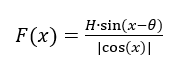(1)

Where H is the intensity of the magnetic field or amplitude of the function, and it is determined by temperature (H) and x denotes the degrees of a circle.

Where is this Formula (1) from? The Formula extraction is described in 2018. [12-14]

The start to open the hysteresis was based on Snell’s law of light refraction.

Figure 2: Algorithm for the opening of ferromagnetic hysteresis a) Traditional hysteresis, b) Reverse hysteresis, c) Left open hysteresis d) Right open hysteresis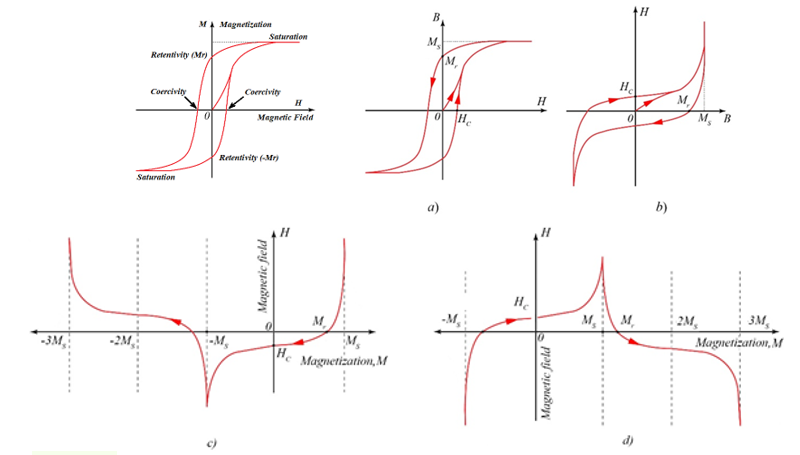The law of superconductivity is nothing but the open hysteresis law of the STE. The horizontal axis shows saturations of the magnetization (polarization, resistivity, temperature, and so on) exhibit the singularities arrowing to infinities.

Equation (2) exhibits the different intensity of the electron ejected and their Fermi-Dirac distributions (Figure 3).

The intensity of the electric current is as next:

Figure 3: The surrounding field of the negative singularity (a)is the left open reverse and (b) is right open hysteresis [12-14]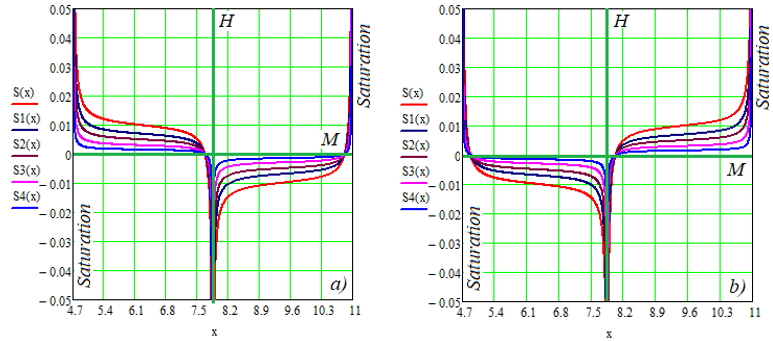When the external magnetic field is active and θ is negative the left side of the open hysteresis shifts downward, and the right-side uplifts as shown in Equation (2) and Figure 3b.

Figure 4: Open hysteresis of the magnetism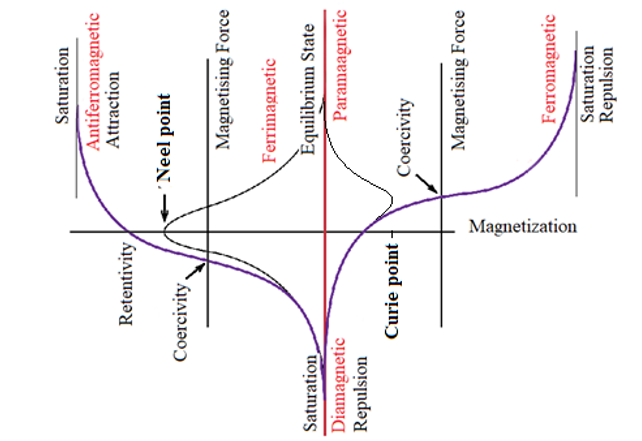Figure 4 exhibits the right-side uplifted hysteresis which includes all the properties of the magnetism. For example, there are ferromagnetic, antiferromagnetic, ferrimagnetic, paramagnetic and diamagnetic in aspects of magnetism. Also, there are ferroelectric, antiferroelectric, and paraelectric. Neel temperature is the temperature above which an antiferromagnetic substance loses its antiferromagnetism and becomes paramagnetic material  while the Curie temperature is known as the temperature at which the ferromagnetic element starts to lose its magnetism and becomes paramagnetic when this temperature is exceeded .

## The Less-Known World Under the Critical Temperature (Tc)

Critical Temperature of the Superconductors

The critical field refers to the maximum magnetic field strength below which the material remains superconducting.  The critical point of superconductivity looks like the event horizon of the black hole horizon or Schwarzchild radius. 

The Schwarzchild solution to Einstein’s field equation (3)  is as follows: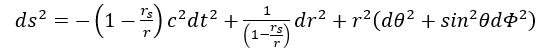(3)

In Equation (3) the Schwarzchild radius is the expression next: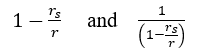(4)

According to the Supreme Theory of Everything, the Schwarzchild radius is only an approximation of the sine function: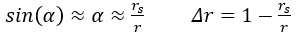(5)

Because of this, we can take an interesting conclusion that Einstein’s field equation becomes more precise if need someone: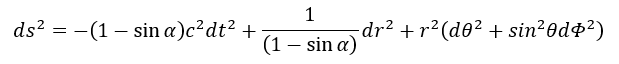The sine function is a dividend of our Formula (1). For this reason, we don’t need to use the Schwarzschild solution for calculating both the event horizon of the black hole and the superconductivity.

We can write the radius of the black hole singularity or the critical temperature in the next trigonometric form: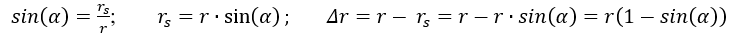(6)

The singularity locates near 270 degrees (Figure (5)).

Figure 5: The negative singularity of the open hysteresis as a critical temperature of the superconductivity (The picture transformed based on )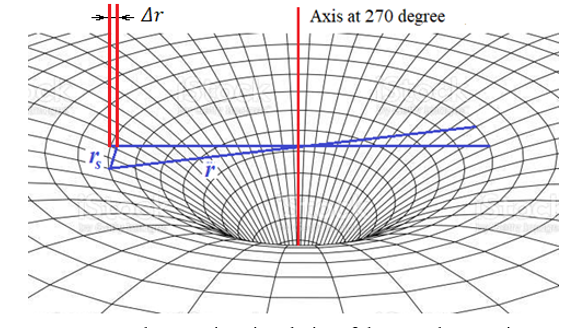The negative singularity shown in Figure 5 illustrates its 3D space near 270 degrees of a circle. The critical temperature (Tc) fluctuates around the 0 K temperature in the unit of a circle. The dependence (Equation (7) and Table 1) between the depth of the singularity (d(x)) and the critical temperature of the superconductivity is as follows: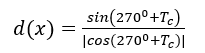(7)

Table 1: The influence depth of critical temperature in a negative singularity.

 Tc d(x) Tc d(x) 20 -2.75 0.01 -5,729.58 15 -3.73 0.005 -11,459.15 10 -5.67 0.0001 -572,957.79 5 -11.47 0.00005 -1,145,915.59 1 -57.29 0.000001 -57,295,779.51 0.5 -114.59 0.0000005 -114,591,559.02 0.1 -572.96 0.00000001 -5,729,577,951.31 0.05 -1145.91 0.000000005 -11,459,155,902.61

To study the microscopic properties of the superconductivity happening in the negative singularity we need to select the region near 0 Kelvin where θ is zero in Formula (1). It means that the external impact on the superconductivity in negative singular is negligible. And in the diamagnetic environment, the non-ohmic resistors become superconductors. So, we need to attend to the short interval surrounding the 0 K temperature (Figure 5).

Classical physics teaches that the I-type superconductor differs from II-type  while STE shows that is indistinguishable. The resistivity in I-type superconductors isn’t refracted rapidly, it is only a curvature in a very short interval (Figure 6).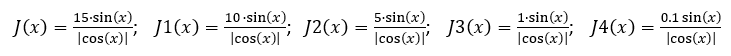(8)

Figure 6: Voltage in the plot of current and resistivity without the external force.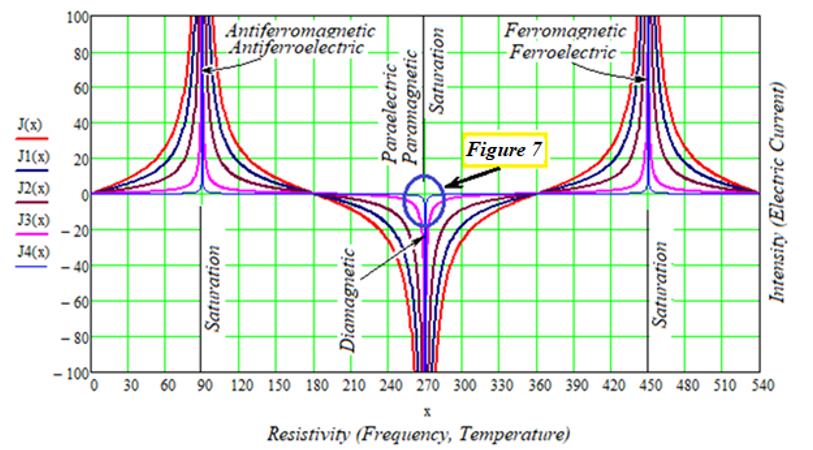The highest temperatures are in the interval between 0-180 degrees, and the lowest temperatures are between 180-360 (0)) degrees. Fermi-Dirac distributions and Debye’s  model play a meaningful role at the interval 90-270 and 270-450 degrees, respectively. The curves of the photoelectric effect in Figure 5 fit with the Fermi-Dirac distribution and the Debye model. The inflection point lies at 0, 180, and 360 degrees. The transition from a normal state to a superconducting, but the transition doesn't continue abruptly and sharply for either type-I superconductor or type-II superconductor.  A system with hysteresis has memory. Its output depends on where it came from. 

We conclude the next:

The 0 Kelvin temperature and the 0 resistivities lie at 270 degrees of a circle. This axis includes the diamagnetic and paramagnetic.  Also, we see that the Fermi-Dirac distribution is between 90-270 and 270-450 degrees. We distinguish the superconductors from I-type superconductors and II-type superconductors because the inflection points of the curves in the I-type superconductor doesn’t been measured by detailed measurement.

Figure 7 includes different non-ohmic resistivity (S(x)), negative singularity, and diamagnetic in the interval of 270°±1° in which Bardeen-Cooper-Schrieffer theory (BCS) works. Here the Cooper-pairs of electrons become the boson and superconductivity, superfluid, and Meissner effects dominate. Since, they, at first, obey Fermi-Dirac statistics due to fermions, but now in the negative singularity of hysteresis (Figure 7) obey the Bose-Einstein distribution due to bosons. The formulas of the Fermi-Dirac and Bose-Einstein distributions are distinguishable only by the interval of a period in a circle (Formula (2) and Formula (9)).

Paramagnetic is a kind of magnetism where several objects are attracted through an externally applied magnetic field. Whereas the diamagnetic materials are repelled by magnetic fields and develop induced magnetic fields in the direction which is opposite to that of the applied magnetic fields.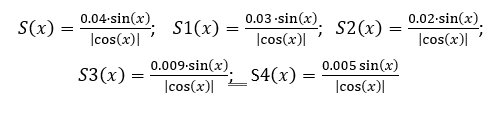(9)

Figure 7: Trigonometric axis (270°) related to 273.15°C (0 K) temperature (Interval between 269° and 271°)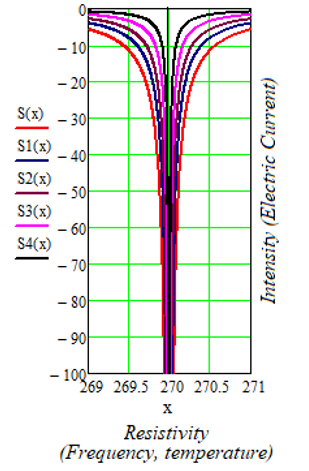The vertical trigonometric axis (270°) is the negative singularity, diamagnetic, and 0 K temperature axis. We see that there is a difference of 3.15°C between the trigonometric axis and the temperature of 0 Kelvin. The origin of this bias is undefined. We know that these are completely different subjects. Anyway, we carry out the calculations based on the trigonometric method in this paper.

Figure 8: Dependence of the drift velocity on temperature  (Red color is measured data and the green color indicates the possible drift velocity according to STE)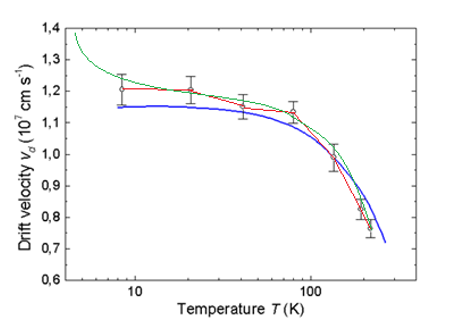From Figure 8, the drift velocity is 100 km/sec, which is not so high. I think the majority of superconductivity begins at lower than minus 10 degrees Kelvin and the electrons accelerate in this interval. I hope it will be connected as shown green-colored curve if we can probe it by experimental.

Figure 9: Example of an experimental electrical resistance response for a type II superconducting material . The response of a type I superconductor is also provided to contrast the sharpness of transition at the critical temperature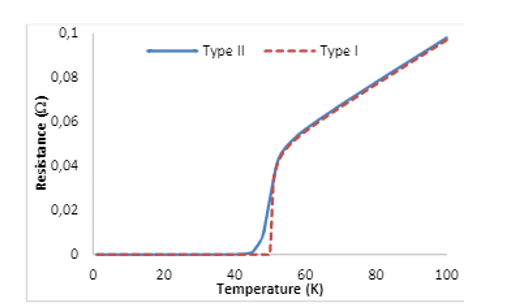The Bose-Einstein distribution is not discreet. The short gap between the two curves (Type II and Type I superconductors in Figure 9) contains a lot of the same curves. So, we don’t separate Type I and Type II (see Figure 6 and Table 1). One law of superconductivity exists in nature, and the type of curve depends on the chemical contents and crystal structures of the given materials.

## Conclusions

1. The superconductivity and nonohmic resistivity can be described by the open hysteresis of the Supreme Theory of Everything as the next:
2. Critical Temperatures of the Superconductors show the material’s lowest resistivities.
3. I and II-type superconductors don’t differ from each other.